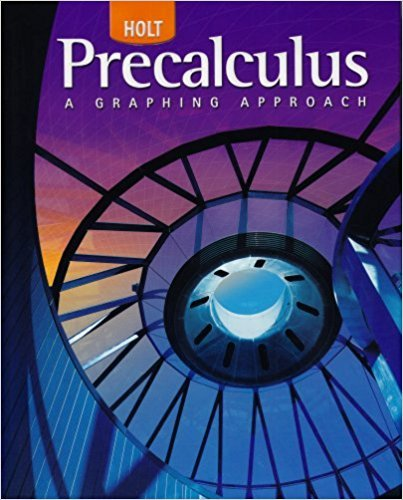×
×

# Solutions for Chapter 11.4.A: Analytic Geometry## Full solutions for Precalculus | 1st Edition

ISBN: 9780030416477Solutions for Chapter 11.4.A: Analytic Geometry

Solutions for Chapter 11.4.A
4 5 0 380 Reviews
18
2
##### ISBN: 9780030416477

Chapter 11.4.A: Analytic Geometry includes 2 full step-by-step solutions. This expansive textbook survival guide covers the following chapters and their solutions. Since 2 problems in chapter 11.4.A: Analytic Geometry have been answered, more than 24517 students have viewed full step-by-step solutions from this chapter. Precalculus was written by and is associated to the ISBN: 9780030416477. This textbook survival guide was created for the textbook: Precalculus, edition: 1.

Key Calculus Terms and definitions covered in this textbook
• Complex number

An expression a + bi, where a (the real part) and b (the imaginary part) are real numbers

• Conversion factor

A ratio equal to 1, used for unit conversion

• Decreasing on an interval

A function f is decreasing on an interval I if, for any two points in I, a positive change in x results in a negative change in ƒ(x)

• Graph of a function ƒ

The set of all points in the coordinate plane corresponding to the pairs (x, ƒ(x)) for x in the domain of ƒ.

• Horizontal Line Test

A test for determining whether the inverse of a relation is a function.

• Intercept

Point where a curve crosses the x-, y-, or z-axis in a graph.

• Inverse variation

See Power function.

• Measure of an angle

The number of degrees or radians in an angle

• Open interval

An interval that does not include its endpoints.

• Opens upward or downward

A parabola y = ax 2 + bx + c opens upward if a > 0 and opens downward if a < 0.

• Phase shift

See Sinusoid.

• Position vector of the point (a, b)

The vector <a,b>.

• Power regression

A procedure for fitting a curve y = a . x b to a set of data.

• Product of complex numbers

(a + bi)(c + di) = (ac - bd) + (ad + bc)i

• Random numbers

Numbers that can be used by researchers to simulate randomness in scientific studies (they are usually obtained from lengthy tables of decimal digits that have been generated by verifiably random natural phenomena).

• Reciprocal of a real number

See Multiplicative inverse of a real number.

• Reflection across the y-axis

x, y and (-x,y) are reflections of each other across the y-axis.

• Solve by substitution

Method for solving systems of linear equations.

• Union of two sets A and B

The set of all elements that belong to A or B or both.

• Window dimensions

The restrictions on x and y that specify a viewing window. See Viewing window.

×